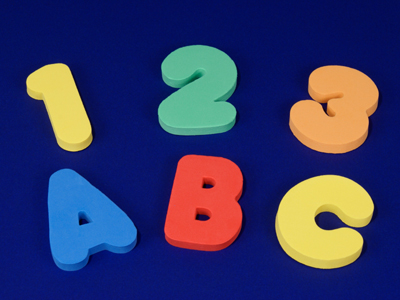Keep checking that you've got the correct letter-number match!

# Verbal Reasoning - Letters for Numbers

This Math quiz is called 'Verbal Reasoning - Letters for Numbers' and it has been written by teachers to help you if you are studying the subject at middle school. Playing educational quizzes is a fabulous way to learn if you are in the 6th, 7th or 8th grade - aged 11 to 14.

It costs only \$12.50 per month to play this quiz and over 3,500 others that help you with your school work. You can subscribe on the page at Join Us

In this quiz, letters stand for numbers. Work out the correct answer to each sum and choose the correct answer from the four choices available. Enjoy but watch out for tricky answers! There's even a little bit of Pythagoras' theorem in this quiz. Don't worry if you aren't familiar with that yet - just enjoy Letters for Numbers!.

Please note that these questions assume you have not yet learned BODMAS, so if you have, please don't use BODMAS!

Example: What is the answer to the sum below, written as a letter?

A = 5, B = 14, C = 12, D = 13, E = 3
A + C - E = ?

A
B
C
E

Answer: The correct answer is B. If we translate all the letters into numbers, our new sum is 5 + 12 - 3, which = 14. 14 = B.
1.
What is the answer to the sum, written as a letter?

V = 32, E = 5, R = 18, Y = 25
E x E + Y - R
V
E
R
Y
If we translate all the letters into numbers, our new sum is 5 x 5 + 25 - 18, which = 32. 32 = V. Interesting number alert! The 5 times tables always end in 5 or 0. 5, 10, 15, 20, 25
2.
What is the answer to the sum, written as a letter?

C = 3, O = 4, D = 5, E = 25
C x C + (O x O) ÷ D
C
O
D
E
If we translate all the letters into numbers, our new sum is 3 x 3 + 4 x 4 ÷ 5, which = 5. 5 = D. Interesting number alert! Squaring the numbers 3 and 4 (9 and 16) and adding them, gives you the square of 5. So 32 + 42 = 52
3.
What is the answer to the sum, written as a letter?

T = 11, I = 99, N = 9, Y = 1
I ÷ T ÷ N x Y
T
I
N
Y
If we translate all the letters into numbers, our new sum is 99 ÷ 11 ÷ 9 x 1, which = 1. 1 = Y. Interesting number alert! The 11 times tables (up to 11 x 11) are all palindromes (they read the same forwards and backwards)
4.
What is the answer to the sum, written as a letter?

S = 22, C = 27, U = 45, N = 5
U ÷ (C - S) x N
S
C
U
N
If we translate all the letters into numbers, our new sum is 45 ÷ (27 - 22) x 5, which = 45. 45 = U
5.
What is the answer to the sum, written as a letter?

H = 3, A = 12, R = 15, D = 2
H + A x D ÷ R
H
A
R
D
If we translate all the letters into numbers, our new sum is 3 + 12 x 2 ÷ 15, which = 2. 2 = U
6.
What is the answer to the sum, written as a letter?

B = 20, U = 4, L = 32, K = 16
L ÷ U + K - B
B
U
L
K
If we translate all the letters into numbers, our new sum is 32 ÷ 4 + 16 - B , which = 4. 4 = U. Interesting number alert! 4 is a very important number in our culture. We have four seasons in a year and four school terms in a school year
7.
What is the answer to the sum, written as a letter?

P = 3, A = 27, L = 6, E = 9
P x E ÷ A x L
P
A
L
E
If we translate all the letters into numbers, our new sum is 3 x 9 ÷ 27 x 6, which = 6. 6 = L
8.
What is the answer to the sum, written as a letter?

M = 5, I = 3, L = 2 , K = 15
K ÷ M + L
M
I
L
K
If we translate all the letters into numbers, our new sum is 15 ÷ 5 + 2, which = 5. 5 = M. Interesting number alert! 2 and 5 are the only prime numbers that end with a 2 or a five
9.
What is the answer to the sum, written as a letter?

B = 5, A = 8, I = 3, T = 6
I + A - T + I
B
A
I
T
If we translate all the letters into numbers, our new sum is 3 + 8 - 6 + 3, which = 8. 8 = A
10.
What is the answer to the sum, written as a letter?

T = 8, Y = 4, R = 12 , E = 2
T x Y ÷ E - R
T
Y
R
E
If we translate all the letters into numbers, our new sum is 8 x 4 ÷ 2 - 12, which = 4. 4 = Y. Interesting number alert! 4 is the only number where the letters that spell it is equal to its value
Author:  Stephen O'Hara